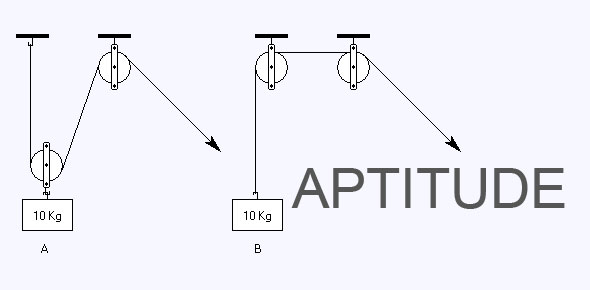# Online Aptitude Test For Developer

15 Questions | Attempts: 276
ShareSettings• 1.
In a certain code language K is written as 11 and KEEP is written as 37. How will the word DRAFT be written in that code language?
• A.

45

• B.

49

• C.

46

• D.

48

• 2.
If Sand is coded as Brick, Brick as House, House as Temple, Temple as Palace then where do you worship?
• A.

Palace

• B.

Temple

• C.

Brick

• D.

House

• 3.
If in code of alphabet AT = 20, BAT = 40 then CAT = ?
• A.

A.34

• B.

B.56

• C.

C.40

• D.

D.60

• 4.
SELECTION BASED ON GIVEN CONDITIONS A few essential criteria for selection of group of items are given: From amongst five doctors A,B,C,D and E;four engineers G,H,K and L; six teachers M,N,O,P,Q and R some teams are to be selected.A,B,G,H,O,P and Q are females and the rest are males. Where ever there is a male doctor ,no female teacher. Where ever there is a male engineer, no female doctor. There shall not be more than two male teachers in any team. If the team consists of 2 doctors, 3 female teachers and 2 engineers, the members of the team are:
• A.

A B O P Q G H

• B.

C D O P Q G H

• C.

C D K L O P Q

• D.

D E G H O P Q

• 5.
Compare the knowledge of persons X, Y, Z, A, B and C in relation to each other 1.X knows more than A. 2.Y knows as much as B. 3.Z knows less than C. 4.A knows more than Y The best knowledge person amongst all is:
• A.

X

• B.

Y

• C.

A

• D.

C

• 6.
Read the following information carefully and answer the questions given below it: (A)Gopal is shorter than Ashok but taller than Kunal. (B)Navin is shorter than Kunal. (C)Jayesh is taller than Navin. (D)Ashok is taller than Jayesh. Who among them is the tallest?
• A.

Gopal

• B.

Ashok

• C.

Kunal

• D.

Navin

• 7.
Arrange the words given below in a meaningful sequence. 1. Protect&nbsp; 2. Pressure 3. Relief 4. Rain 5. Flood
• A.

2, 4, 3, 1, 5

• B.

2, 4, 5, 1, 3

• C.

2, 5, 4, 1, 3

• D.

3, 2, 4, 5, 1

• 8.
Select the pair of words which has the same relationship. ARMY:LOGISTICS
• A.

• B.

Soldier:Students

• C.

War:Logic

• D.

Team:Individual

• 9.
In an arithmetic series consisting of 51 terms, the sum of the first three terms is 65 and the sum of the middle three terms is 129. What is the first term and the common difference of the series?
• A.

64, 9/8

• B.

32, 8/9

• C.

187/9, 8/9

• D.

72, 9/8

• 10.
If Sn denotes the sum of the first n terms in an Arithmetic Progression and S1: S4 = 1: 10 Then the ratio of first term to fourth term is:
• A.

1: 3

• B.

2: 3

• C.

1: 4

• D.

1: 5

• 11.
The total capital investment in setting up a production unit is Rs.15 lakhs and the production unit produces 3 million kg of product annually. Selling price of product is 9 per kg. Manufacturing cost include raw material at the rate Rs.0.8 per kg, labour at the rate 1.2 per kg, packing at the rate of 0.6 per kg and utilities at the rate of 0.8 per kg. General expenses comes out to be 15% of manufacturing cost. If taxes are considered as 33% of gross annual earnings, determine the net profit of the production unit per year?
• A.

0.5 crores

• B.

1.02 crores

• C.

1.5 crores

• D.

2 crores

• 12.
Six bells commence tolling together and toll at intervals of 2, 4, 6, 8 10 and 12 seconds respectively. In 30 minutes, how many times do they toll together?
• A.

4

• B.

10

• C.

15

• D.

16

• 13.
The square of a two digit number is divided by half the number. After 36 is added to the quotient, this sum is then divided by 2. The digits of the resulting number are the same as those in the original number, but they are in reverse order. The ten`s place of the original number is equal to twice the difference between its digits. What is the number?
• A.

45

• B.

46

• C.

47

• D.

48

• 14.
Two merchants, M and N, enter into partnership. M puts in Rs. 20,000 in the first month and at the end of four months adds Rs.5,000 to his capital. While N withdraws Rs.20, 000 at the end of 8 months. At the end of the year, M and N received equal profit. Find the amount invested by N ?
• A.

Rs.20,000

• B.

Rs.30,000

• C.

Rs.40,000

• D.

Rs.50,000

• 15.
A,B and C enter into a partnership by investing in the ratio of 3:2:4. After one year ,B invests another Rs 2,70,000 and C,at the end of 2 years, also invests Rs 2,70,000.At the end of 3 years ,profit are shared in the ratio of 3:4:5.Find the initial investment of each A?
• A.

2,70,000

• B.

1,80,000

• C.

3,60,000

• D.

None of these

## Related TopicsBack to top
×

Wait!
Here's an interesting quiz for you.